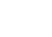Benchmark Replication via Dimension Reduction

Our algorithms are able to track the performance of any target instrument (e.g. index or chosen combination of assets). Here we show you how. Well... not exactly how. But read on!

Background & Introduction

A common endeavor in financial markets is to (attempt to) closely track a reference index. This could be for obvious reasons in the case of an index tracker, or perhaps less obviously in the case of thematic portfolios.

An unrealistically ideal solution for asset or portfolio managers would be to somehow efficiently buy/sell exactly the same stocks that mirror the index - however this approach is bound to face a dual challenge of low liquidity and high trading costs.

In this insight, we'll demonstrate that we can accurately track the performance of a large composite index using dimension reduction algorithms, thus producing a well performing tracking portfolio.

Approach

Assuming one cannot efficiently buy/sell the same stocks that constitute the entire index, an attractive but somewhat complex alternative is to reduce the dimension of the universe by finding a subset of tradable assets that can capture most of the characteristics (in this case volatility) of the target index. This can be achieved using a variety of clustering and dimension reduction techniques.

Once a smaller group of assets is identified that displays said characteristics, we can then try to minimize the tracking error of such a portfolio holding this subset of assets vs. the target index.

In the example below we will apply a dimension reduction methodology to create a synthetic fund that tracks the Dow Jones Islamic Market (DJIM) Index. For reference, the DJIM is composed of around 2,500 stocks that pass rules-based screens for adherence to Sharīʿah investment guidelines.

Our initial objective will be to reduce the dimensionality of the problem, finding 50 stocks that capture enough of the volatility from the index to create a viable strategy.

Stock Picking Method

A dimension reduction methodology will be applied to the covariance matrix of the universe of stocks. Using this approach we will produce a variety of clusters that will ideally capture different risk characteristics of the universe of stocks.

In order to back out the specific stocks we will use the relative importance of the clusters and the importance of each stock within its cluster.

Index Tracking Method

Once we have defined a universe of stocks, we will then run a regression to calculate the weights of each stock in the synthetic portfolio and invest in that universe the following day.

We will run this process daily with a training set size of 400 days. We will then apply this methodology on a rolling basis.

Example 1

We show the tracking results as well as a linear regression between the target index and the strategy:

• Regularization parameter = 5
• Number of clusters = 5

By running this configuration, we get an adjusted R-squared of 99.3%.

Example 2

We show the tracking results as well as a linear regression between the target index and the strategy. In this example we modified the regularization parameter in the clustering algorithm.

• Regularization parameter = 10
• Number of clusters = 5

By running this configuration, we get an adjusted R-squared of 98.8%.